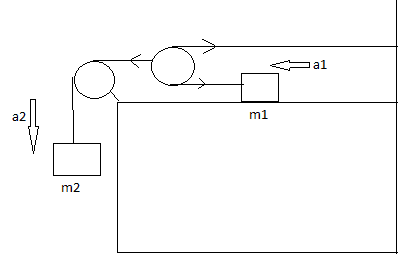# Relationship of acceleration in two-pulley system

## Homework StatementWhat is the relationship between a1 and a2
A.a1=a2
B.a1=2a2
C.a2=2a1
D.a1=a2√2
E.a2=a1√2
F.There is no simple relationship between the two acceleration

a = F/m

## The Attempt at a Solution

I am quite confused with the second pulley , so the weight of m2 is divided to 2 tension through the second pulley , but i cant find what is the magnitude of the upper tension ,therefore we cant find the lower tension or the acceleration either.
So i choose F as my answer , however i am not sure . Is my answer right ?.

Last edited:

DEvens
Gold Member
Conserve string.

Conserve string.

sorry i dont get it . can you be more specific , and which answer do you choose :D

DEvens
Gold Member
Really? That's not enough?

Ok. When mass m1 moves a distance of 1 unit, how far must the mass m2 move?

That's where i was confused.
If m2 goes a distance of x. Will m1 goes a distance of x/2 or 2x?

haruspex
Homework Helper
Gold Member
That's where i was confused.
If m2 goes a distance of x. Will m1 goes a distance of x/2 or 2x?
Let the lengths of the horizontal portions be x (on the left), y (top right), z (lower right).
What equations relate them?

I have the same problem too
Let the lengths of the horizontal portions be x (on the left), y (top right), z (lower right).
What equations relate them?

I think that it is Δx = Δy + Δz
Since Δy is the same as Δz, so Δx = 2Δy = 2Δz
So, a2 = 2a1, right ?

BvU
Homework Helper
Try again.

Try again.
Hmm..
I tried and got the same answer, a2 = 2a1

EDIT :
Tried again and get a1 = 2a2

Last edited:
haruspex
Homework Helper
Gold Member
Tried again and get a1 = 2a2
Good.

•terryds
Hmm..
I tried and got the same answer, a2 = 2a1

EDIT :
Tried again and get a1 = 2a2

sr i didnt understand , since S = 1/2 at^2
then if Δx=2Δy .
then ax should be equal 2 ay ?
then a2 = 2a1 ?

haruspex